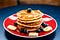# Reverse Polish notation is easy with test-driven development“baked pancake with blueberry and slice of banan” by nikldn on Unsplash. I chose this one mostly because it’s a stack of pancakes, and thus not completely irrelevant to the topic at hand.

# Background: the need for reverse Polish notation

`mpg = endMileage - startMileage / gallonsUsed;`

# Writing the tests for a reverse Polish calculator in Java

`public class ReversePolishCalculator {    public String history() {        return "This feature has not been implemented yet.";    }    public double calculate(String expression) {        return 0.0;    }}`
`    @Test    public void testHistory() {        System.out.println("history");        String expResult = "";        String result = revPolCalc.history();        assertEquals(expResult, result);        String expression = "1 1 +";        revPolCalc.calculate(expression);        expResult = expression + "\n= 2\n";        result = revPolCalc.history();        assertEquals(expResult, result);        expression = "1 2 3 * - 4 /";        revPolCalc.calculate(expression);        expResult = expResult + "\n" + expression + "\n= -1.25\n";        result = revPolCalc.history();        assertEquals(expResult, result);    }`
`    @Test    public void testCalculate() {        System.out.println("calculate");        String expression = "1 1 +";        double expResult = 2.0;        double result = revPolCalc.calculate(expression);        assertEquals(expResult, result, TEST_DELTA);        expression = "1 3 /";        expResult = 0.33333;        result = revPolCalc.calculate(expression);        assertEquals(expResult, result, TEST_DELTA);    }`
`    @Test    public void testCalculateOnGivenExpression() {        String expression = "3 2 + 8 4 * -";        System.out.println("calculate \"" + expression + "\"");        double expResult = -27.0;        double result = revPolCalc.calculate(expression);        assertEquals(expResult, result, TEST_DELTA);    }`
`    /**     * Test of calculate method, of class ReversePolishCalculator.     * The obelus ("&divide;", Unicode 0x00F7) should work the same     * as the forward slash ("/", ASCII 0x2F).     */    @Test    public void testCalculateWithObelus() {        String expression = "1 7 \u00F7";        String exprWSlash = expression.replace("\u00F7", "/");        System.out.println("calculate \"" + expression + "\" should work the same as " + exprWSlash);        double expResult = 0.142857;        double result = revPolCalc.calculate(expression);        assertEquals(expResult, result, TEST_DELTA);        double resultWSlash = revPolCalc.calculate(exprWSlash);        assertEquals(result, resultWSlash, TEST_DELTA);    }`

# Starting to write the implementation of a reverse Polish calculator in Java

`scala> var expression = "Hello world! This is a String."expression: String = Hello world! This is a String.scala> expression.split(" ")res0: Array[String] = Array(Hello, world!, This, is, a, String.)scala> expression = "3 2 + 8 4 * -"expression: String = 3 2 + 8 4 * -scala> expression.split(" ")res1: Array[String] = Array(3, 2, +, 8, 4, *, -)`
`    public double calculate(String expression) {        Stack<String> stack = new Stack();        String[] elems = expression.split(" ");        double operand1, operand2;        for (String elem : elems) {            stack.push(elem);        }         // Now we parse, right?        return 0.0; // Keeping this line for now    }`
`    public double calculate(String expression) {        Stack<Double> stack = new Stack();        String[] elems = expression.split(" ");        boolean numParseFlag;        char currChar;        double operand1, operand2, parsedNum;        double currVal = 0.0;        for (String elem : elems) {            numParseFlag = true;            if (elem.length() == 1) {                numParseFlag = false;                currChar = elem.charAt(0);                switch (currChar) {                    case '+':                        operand1 = stack.pop();                        operand2 = stack.pop();                        currVal = operand1 + operand2;                        stack.push(currVal);                        break;                    case '-':                        operand1 = stack.pop();                        operand2 = stack.pop();                        currVal = operand1 - operand2;                        stack.push(currVal);                        break;                    case '*':                        operand1 = stack.pop();                        operand2 = stack.pop();                        currVal = operand1 * operand2;                        stack.push(currVal);                        break;                    case '/':                        operand1 = stack.pop();                        operand2 = stack.pop();                        currVal = operand1 / operand2;                        stack.push(currVal);                        break;                    default:                        numParseFlag = true;                }            }            if (numParseFlag) {                try {                    parsedNum = Double.parseDouble(elem);                    stack.push(parsedNum);                } catch (NumberFormatException nfe) {                    throw nfe;                }            }        }        return currVal;    }`
`Testcase: testCalculateWithObelus(basicexercises.ReversePolishCalculatorTest): Caused an ERRORFor input string: "÷"java.lang.NumberFormatException: For input string: "÷" ...Testcase: testCalculate(basicexercises.ReversePolishCalculatorTest): FAILEDexpected:<0.33333> but was:<3.0> ...Testcase: testCalculateWithMinusSign(basicexercises.ReversePolishCalculatorTest): Caused an ERRORFor input string: "?"java.lang.NumberFormatException: For input string: "?" ...Testcase: testCalculateOnGivenExpression(basicexercises.ReversePolishCalculatorTest): FAILEDexpected:<-27.0> but was:<27.0> ...Testcase: testHistory(basicexercises.ReversePolishCalculatorTest): FAILEDexpected:<[]> but was:<[This feature has not been implemented yet.]> ...Testcase: testDivisionByZeroCausesException(basicexercises.ReversePolishCalculatorTest): FAILEDCalculating "0 0 /" should have triggered an exception, not given result NaN. ...Testcase: testCalculateWithMultCross(basicexercises.ReversePolishCalculatorTest): Caused an ERRORFor input string: "×"java.lang.NumberFormatException: For input string: "×" ...`
`                    case '-':                        operand2 = stack.pop();                        operand1 = stack.pop();                        currVal = operand1 - operand2;                        stack.push(currVal);                        break;                    ...omitting case '*', no change...                    case '/':                        operand2 = stack.pop();                        operand1 = stack.pop();                        currVal = operand1 / operand2;                        stack.push(currVal);                        break;`
`                    case '-':                    case '\u2212':                        operand2 = stack.pop();                        operand1 = stack.pop();                        currVal = operand1 - operand2;                        stack.push(currVal);                        break;                    case '*':                    case '\u00D7':                        operand2 = stack.pop();                        operand1 = stack.pop();                        currVal = operand1 * operand2;                        stack.push(currVal);                        break;                    case '/':                    case '\u00F7':                        operand2 = stack.pop();                        operand1 = stack.pop();                        currVal = operand1 / operand2;                        stack.push(currVal);                        break;`
`    public double calculate(String expression) {        calcHistory = calcHistory + expression + "\n";        Stack<Double> stack = new Stack();        String[] elems = expression.split(" ");        ...omitting several lines quoted above...                parsedNum = Double.parseDouble(elem);                stack.push(parsedNum);            }        }        calcHistory = calcHistory + "= " + currVal;        if (calcHistory.endsWith(".0")) {            calcHistory = calcHistory.substring(0,                                          calcHistory.length() - 2);        }        calcHistory = calcHistory + "\n";        return currVal;    }    }`
`Calculating "0 0 /" should have triggered an exception, not given result NaN.`
`scala> var numberA = Math.sqrt(2)numberA: Double = 1.4142135623730951scala> val zero = 0.0zero: Double = 0.0scala> numberA / zerores2: Double = Infinityscala> numberA = numberA * -1numberA: Double = -1.4142135623730951scala> numberA / zerores3: Double = -Infinity`
`scala> numberA.isNaNres4: Boolean = false`
`scala> res2.isNaNres5: Boolean = falsescala> res3.isNaNres6: Boolean = false`

# The next steps

`                        operand2 = stack.pop();                        operand1 = stack.pop();`

Written by